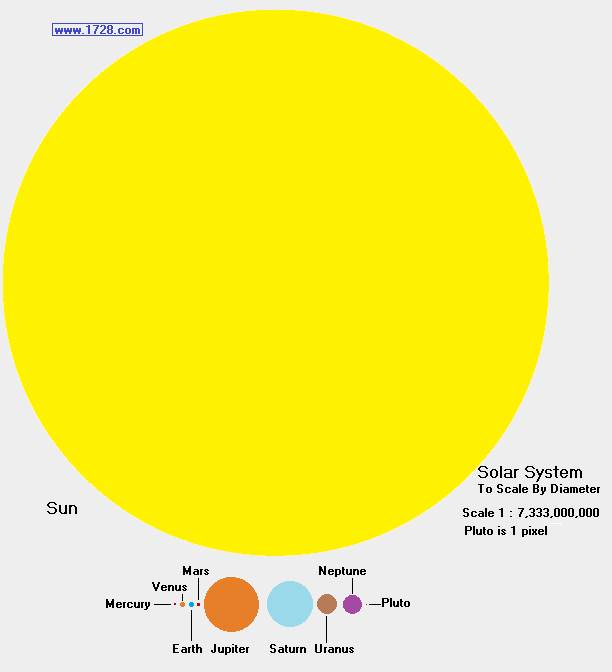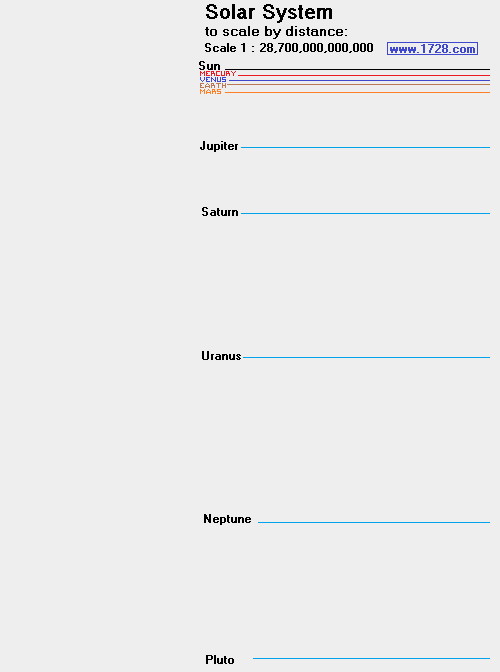Solar System Calculator
Scroll to the bottom of the page to read "An Impressive Solar System Model".   A scale model with quite a difference.

 To construct a solar system model, enter 5 (for example) in the scale factor box, click "Earth diameter" and you will have all the dimensions in terms of the Earth's diameter. So, a solar system with a 5 inch Earth would have a Sun that is (look at the calculations) 546.49 inches (45.5 feet) in diameter and the Earth to Sun distance would be 58,703 inches (4,892 feet) - almost a mile! Instead, let's try a solar system in which the Sun's diameter equals 5 inches, which means the Earth is 0.045746 inches (about 1.1 mm) wide and the Earth Sun distance would be 537.09 inches (roughly 45 feet). Do you see how difficult it is to construct a solar system model?

 Input Scale Factor >>>Before constructing a solar system model, it is a good idea to have a rough estimate of the diameters and distances. Roughly speaking, if Earth's diameter = 1 Jupiter's diameter = 10 Sun's diameter = 100 Earth to Sun distance = 10,000 Saturn to Sun distance = 100,000 ********************************************************************* An Impressive Solar System Model Here's a model that seems very easy yet is nearly impossible to construct. Let's suppose you want to show Earth in relation to the nearest star α Centauri. Gee? Only two objects to construct? That's got to be easy. Make the Earth just 5 inches in diameter and on this scale α Centauri needs to be a sphere (or to make it a little easier) a circle that is 56 feet in diameter! Okay that is a bit clumsy but the next step is really difficult. And where does this 56 foot model of α Centauri have to be in order for it to be on the same scale as the 5 inch Earth? The Moon !! Yes, a 5 inch scale model of the Earth requires the scale model of the nearest star to be on the Moon if it is to be in scale with the Earth! Just think - this could be an impressive science project. If you are going to have your project judged when the Moon is visible from your classroom, this would really impress the judges and the students!

Significant Figures >>>
Numbers are displayed in scientific notation with the amount of significant figures you specify. For easier readability, numbers between .001 and 1,000 will not be in scientific notation but will still have the same precision.
You may change the number of significant figures displayed by changing the number in the box above.
Most browsers, will display the answers properly but if you are seeing no answers at all, enter a zero in the box above, which will eliminate all formatting but at least you will see the answers.